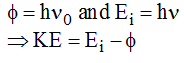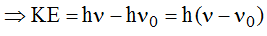# Electromagnetic Energy - Atomic Structure Chemistry Notes | EduRev

## Chemistry : Electromagnetic Energy - Atomic Structure Chemistry Notes | EduRev

The document Electromagnetic Energy - Atomic Structure Chemistry Notes | EduRev is a part of the Chemistry Course Physical Chemistry.
All you need of Chemistry at this link: Chemistry

Electromagnetic Energy

Newton was first person to comment on the nature of light in terms of Corpuscular. Theory of Light. According to this theory light is a stream of particles commonly known as corpuscles of light. He was able to explain reflection and refraction, the most common phenomenon of light. But the other phenomenon like diffraction and interference could not be explained on the basis of this theory.

Maxwell, in 1956 proposed that radiant energy (light) has wave characteristics. Light according to him is Electromagnetic Wave arising due to the disturbance created by electric and magnetic fields, oscillating perpendicular to each other in space. Like all other mechanical waves, it is characterized by velocity c, frequency ν, wavelength λ which are related as:

c = νλ. The value of c is constant and equal to 3 × 108 m/s.

Electromagnetic Spectrum

Electromagnetic wave or radiation is not a single wavelength radiation, but a mixture of various wavelength or frequencies. All the frequencies have same speed.

If all the components of Electromagnetic Radiation (EMR) are arranged in order of decreasing or increasing wavelengths or frequencies, the pattern obtained is known as Electromagnetic Spectrum. The following table shows all the components of light.

 S. No. Name Wavelength Frequency(Hz) Source 1. Radio wave 3×1014 – 3×107 1×105 – 1×109 Alternating current of high frequency 2. Microwave 3×107 – 6×106 1×109 – 5×1011 Klystron tube 3. Infrared (IR) 6×106 – 7600 5×1011 – 3.95×1016 Incandescent objects 4. Visible 7600 – 3800 3.95×1016 – 7.9×1014 Electric bulbs, sun rays 5. Ultraviolet (UV) 3800 – 150 7.9×1014 – 2×1016 Sun says, are lamps with mercury vapours 6. X-rays 150 – 0.1 2×1016 – 3×1019 Cathode rays striking metal plate 7. Γ-Rays 0.1 – 0.01 3×1019 – 3×1020 Secondary effect of radioactive decay 8. Cosmic Rays 0.01 – zero 3×1020 – Infinity Outer space

Photoelectric Effect

It was observed by Hertz and Lenard around 1880 that when a clean metallic surface is irradiated by monochromatic light of proper frequency, electrons are emitted from it. This phenomenon of ejection of the electrons from metal surface was called as Photoelectric effect.

It was observed that if the frequency of incident radiation is below a certain minimum value (threshold) frequency),no emission takes place however high the intensity of light may be.

Another important feature observed was that the kinetic energy of the electrons emitted is independent of the Intensity of the light. The kinetic energy of the electrons increases linearly with the frequency of incident light radiation. This was highly contrary to the laws of Physics at that time i.e., the energy of the electrons should have been proportional to the intensity of the light, not on the frequency.

These features could not be properly explained on the basis of Maxwell’s concept of light i.e. light as electromagnetic wave.

In 1905, Einstein applied Planck’s quantum theory of light to account for the extraordinary features of the photoelectric effect. He introduced a new concept that light shows dual nature. In phenomenon like reflection, refraction and diffraction it shows wave nature and in phenomenon like photoelectric effects, it shows particle nature. According to the particle nature, the energy of the light is carried in discrete units whose magnitude is proportional to the frequency of the light wave. These units were called as photons (or quanta).

According to Einstein, when a quantum of light (photon) strikes a metal surface, it imparts its energy to the electrons in the metal. In order for an electron to escape from the surface of the metal, it must overcomes the attractive force of the positive ions in the metal. So a part of the photon’s energy is absorbed by the metal surface to release the electron, this is known as work function of the surface and is denoted by φ. The remaining part of the energy of the photon goes into the kinetic energy of the electron emitted. If E is the energy of the photon, KE is the kinetic energy of the electron and φ be the work function of the metal then we have;Also, if m be the mass and v be the velocity of the electron ejected then

KE = 1/2  mv2 = h(ν – ν0).

Offer running on EduRev: Apply code STAYHOME200 to get INR 200 off on our premium plan EduRev Infinity!

## Physical Chemistry

77 videos|83 docs|32 tests

,

,

,

,

,

,

,

,

,

,

,

,

,

,

,

,

,

,

,

,

,

;